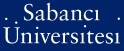The dynamic programming equation for the problem of optimal investment under capital gains taxes

Ben-Tahar, Imen and Soner, Halil Mete and Touzi, Nizar (2007) The dynamic programming equation for the problem of optimal investment under capital gains taxes. (Accepted/In Press)Preview
PDF - Requires a PDF viewer such as GSview, Xpdf or Adobe Acrobat Reader
268Kb

Abstract

This paper considers an extension of the Merton optimal investment problem to the case where the risky asset is subject to transaction costs and capital gains taxes. We derive the dynamic programming equation in the sense of constrained viscosity solutions. We next introduce a family of functions, which converges to our value function uniformly on compact subsets, and which is characterized as the unique constrained viscosity solution of an approximation of our dynamic programming equation. In particular, this result justifies the numerical results reported in the accompanying paper by the authors.

Item Type: Article H Social Sciences > HG FinanceQ Science > QA Mathematics 7100 Halil Mete Soner 16 Nov 2007 10:21 16 Nov 2007 10:21

Available Versions of this Item

Repository Staff Only: item control page